Factoring - Numerical Examples MathBitsNotebook.com Terms of Use   Contact Person: Donna RobertsNumerical factoring is the easiest type of factoring because it simply makes a number look like a multiplication problem. In the following examples, we will be looking at examples involving factoring integers.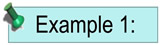Factor 24.

If we do simple integer factorization, we can get a series of answers.
24 = 1 x 24                24 = 2 x 12                24 = 3 x 8                24 = 4 x 6
and of course the order can be reversed (by the commutative property)
24 = 24 x 1                24 = 12 x 2                24 = 8 x 3                24 = 6 x 4

These answers look like listings you saw in a multiplication table when you were first learning to multiply. So which one is the answer?

ANSWER: Actually any one, or all, of these listings are the answer.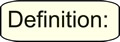Integer factorization is the breaking down of a composite (not prime) number into a product of smaller integers. No specification is necessarily made that the smaller integers must be primes.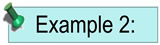Find the prime factors of 24?

This problem is a more specific version of Example 1. In this problem, you are being specifically asked for the prime factors of 24, where each of the factors is a prime number. This problem is asking you to perform a prime factorization.

 The easiest way to find prime factors is to use a factor tree. Start by dividing the smallest possible prime. Continue the process until all prime factors have been found.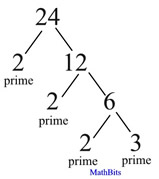A short hand notation of a factor tree is a divisional-style listing such as: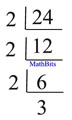ANSWER: The prime factors are 2 x 2 x 2 x 3 or 23 x 3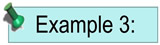What is the prime factorization of 54?
Remember that a prime factorization is looking for only the prime factors of the number.

 Using a factor tree.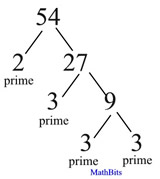Using the short hand notation: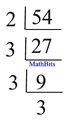ANSWER: The prime factors are 2 x 3 x 3 x 3 or 2 x 33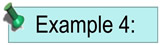Factor the numerical expression 24 + 60.

This problem is a little different than just asking for the factors of a number. In this problem, we will be looking for the largest factor (the GCF) that is shared by 24 and 60. We will then "pull out" this largest factor and place it in front of a set of parentheses surrounding the sum of the remaining factors.

Looking at a prime factorization of these two numbers will show us what the greatest common factor will be for 24 and 60.

 The prime factorization shows us what we have to work with: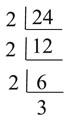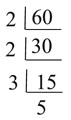Find the prime factors that are shared by both values: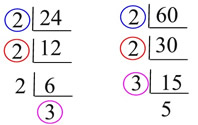The shared prime factors are 2, 2 and 3, making the GCF 2 x 2 x 3 = 12 Now, we need : 24 = 12 x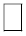and 60 = 12 xNotice that the remaining (unused) prime factors in each of the previous charts will show us the values for the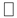s. 24 = 12 x 2 and 60 = 12 x 5. If there is more than one "unused" prime factor left in a chart (or tree), use the product of those unused primes to fill the. Pull the 12 out front and write the sum of the missingvalues in the parentheses. We now have our solution which is: ANSWER: 24 + 60 = 12(2 + 5) Use the distributive property to check the answer: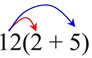Distribute the 12 across the parentheses. 12 x 2 = 24 and 12 x 5 = 60

When we examine the use of the distributive property, in this manner,
we can see that "factoring an expression" can be seen as the opposite of
"distributing (expanding) an expression".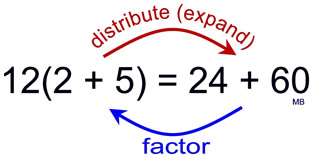This concept will be particularly useful in Algebra, with expressions such as:
12x - 36 = 12(x - 3)This connection between factoring and the distributive property can also be seen
when dealing with area. First let's refresh a few geometry concepts that will be needed.
Consider the following concepts: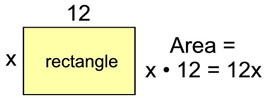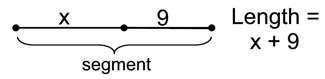Now, examine the following,
where the green "box" represents a rectangle in which the dashed line forms two rectangles: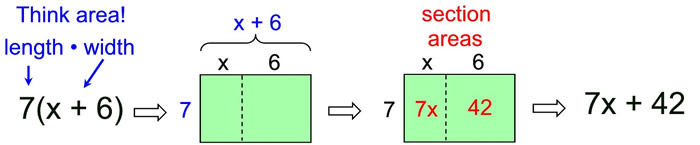This is a visual representation of the distributive property 7(x + 6) = 7x + 42.

It can also be applied in reverse to demonstrate factoring.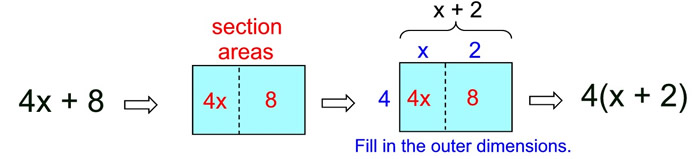In these examples, we have seen area represented as a product, 7(x + 6) and 4(x + 2),
and area represented as a sum, 7x + 42 and 4x + 8.